Next: Orthogonality and Normalization Up: Conserved Current and Charge Previous: Conserved Current and Charge

## Charge of a Klein-Gordon Particle

If two real wave functions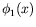and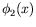separately satisfy a Klein-Gordon equation with the same mass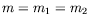, then the separate Klein-Gordon equations can be replaced by one Klein-Gordon equation for a complex wave function: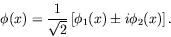(4.50)

Using the definition of charge in equation 4.49, we see that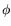and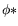have opposite charge and that a real wave function has zero charge. In general we have

 complex scalar fields are charged, real scalar fields are uncharged.

We now examine the charge for a superposition of positive-energy and superposition of negative-energy solutions to the Klein-Gordon equation. If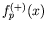is a positive-energy solution to the Klein-Gordon equation with momentum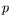, the superposition of all such positive-energy solutions is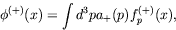(4.51)

where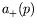is a weighting function of three-momentum only andis given by equation 4.19. The charge for this general positive-energy solution is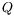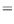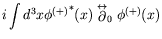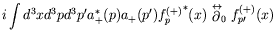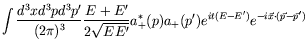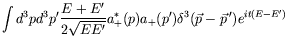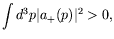(4.52)

where the last step follows because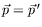implies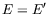.

For a superposition of negative-energy solutions,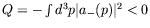. Thus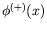specifies a particle with positive charge and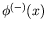a particle with the same mass but negative charge.

For zero charge spin-0 particles, the wave function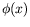must be real: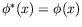. For a single momentum we can write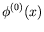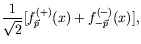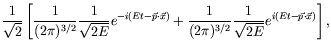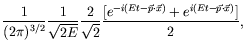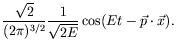(4.53)

From the relativistic wave equation for spin-0 particles and the interpretation of its wave functions, we are lead to three solutions which correspond to the electric charges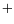,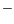and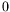for every momentum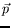. The relativistic quantum theory thus reveals the charge degree of freedom of particles.

According to our current understanding, there are no fundamental spin-0 particles. However, if we do not probe the internal structure of mesons they can be considered as Klein-Gordon particles. For the pion system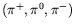with the approximation that each state has the same mass, we write (by convention)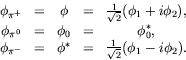(4.54)

By convention it is the positive-charged boson which is the particle and the negative-charge fermion which is the particle.Next: Orthogonality and Normalization Up: Conserved Current and Charge Previous: Conserved Current and Charge
Douglas M. Gingrich (gingrich@ ualberta.ca)
2004-03-18